# Union Clause in SQL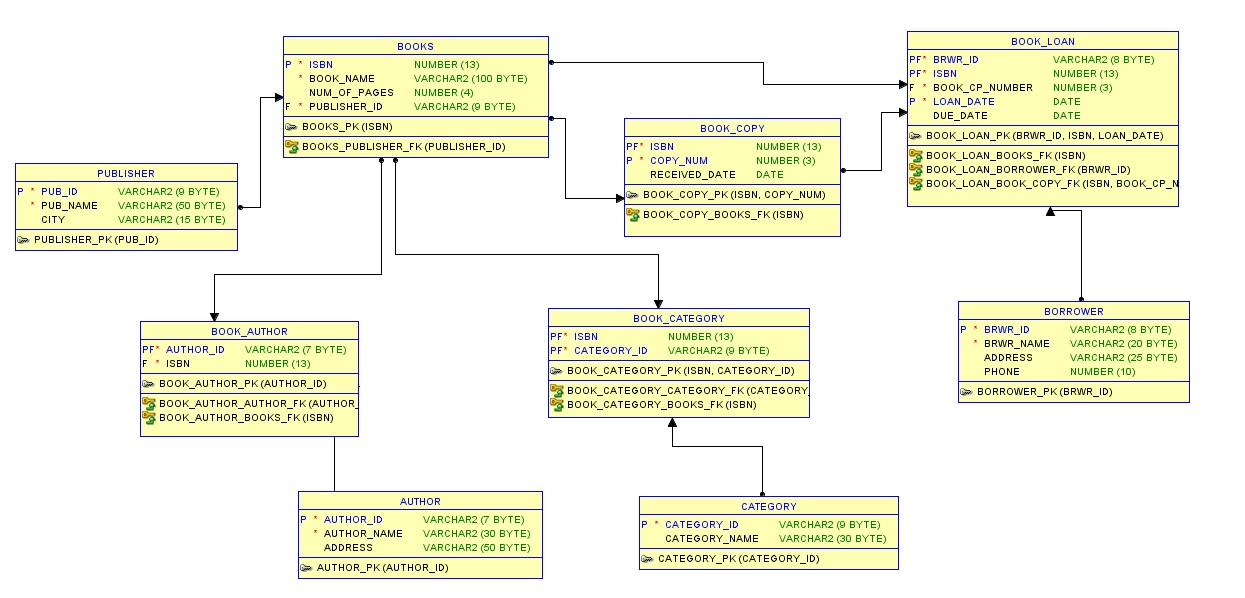Figure 1: Library Database

## Union Clause

Union clause is used to combine the result set of two equivalent queries. That means when two equivalent queries are executed, it results in its own result sets. If we want to see the combined results of them, then we can use UNION to merge the results.

Consider below two queries on BOOK_COPY to retrieve details about two different books. When they are executed separately, it will result in two different sets. But when UNION is used, it combines the results into one.

```SELECT * FROM BOOK_COPY WHERE ISBN = 82014300
UNION
SELECT * FROM BOOK_COPY WHERE ISBN = 9789350871249;```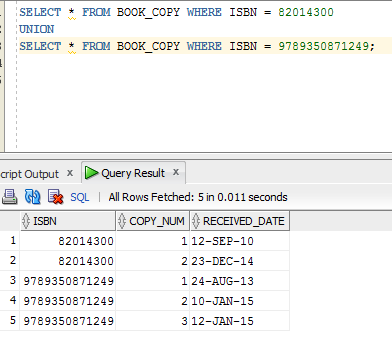While using UNION clause, care should be taken to have same number, datatype and size of the columns in both the queries. We can have different conditions in WHERE clause, but SELECT lists perfectly match with each other. We can even have any number of tables participating in either of query. They need not be same.

```SELECT b.ISBN, b.Book_NAME, bc.CATEGORY_ID, b.PUBLISHER_ID
FROM BOOKS b, BOOK_CATEGORY bc
WHERE b.ISBN = bc.ISBN
AND bc.CATEGORY_ID = 'DBMS_1000'
UNION
SELECT b.ISBN, b.Book_NAME, bc.CATEGORY_ID, b.PUBLISHER_ID
FROM BOOKS b, BOOK_CATEGORY bc
WHERE b.ISBN = bc.ISBN
AND bc.CATEGORY_ID = 'DBMS_1004';```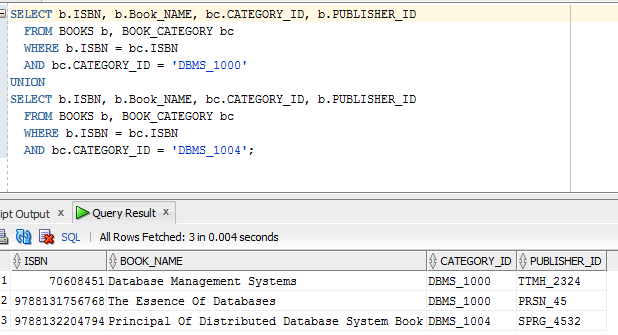When UNION clause is used it eliminates any duplicate records in the result set. In below query, we have records for DBMS_1004 from both the queries. But union clause has eliminated the duplicate value and showed it only once in the result.

```SELECT b.ISBN, b.Book_NAME, bc.CATEGORY_ID, b.PUBLISHER_ID
FROM BOOKS b, BOOK_CATEGORY bc
WHERE b.ISBN = bc.ISBN
AND bc.CATEGORY_ID IN ('DBMS_1004')
UNION
SELECT b.ISBN, b.Book_NAME, bc.CATEGORY_ID, b.PUBLISHER_ID
FROM BOOKS b, BOOK_CATEGORY bc
WHERE b.ISBN = bc.ISBN
AND bc.CATEGORY_ID IN (‘DBMS_1004','ECNM_1101');```

If we want to retain the duplicate values or all the results from both the queries, then we have to use UNI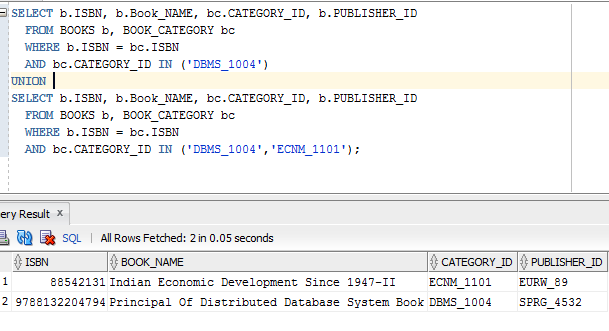If we want to retain the duplicate values or all the results from both the queries, then we have to use UNION ALL clause.

```SELECT b.ISBN, b.Book_NAME, bc.CATEGORY_ID, b.PUBLISHER_ID
FROM BOOKS b, BOOK_CATEGORY bc
WHERE b.ISBN = bc.ISBN
AND bc.CATEGORY_ID IN ('DBMS_1004')
UNION ALL
SELECT b.ISBN, b.Book_NAME, bc.CATEGORY_ID, b.PUBLISHER_ID
FROM BOOKS b, BOOK_CATEGORY bc
WHERE b.ISBN = bc.ISBN
AND bc.CATEGORY_ID IN (‘DBMS_1004','ECNM_1101');```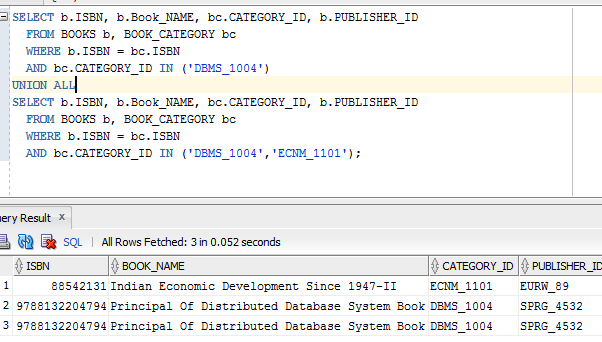Translate »